# Label Semantic Roles¶

The source code of this tutorial is in book/label_semantic_roles. For the new users to Paddle book, please refer to Book Documentation Instructions .

## Background¶

Natural language analysis techniques are roughly divided into three levels: lexical analysis, syntactic analysis, and semantic analysis. Labeling semantic roles is a way to implement shallow semantic analysis. In a sentence, the predicate is a statement or explanation of the subject, pointing out “what to do”, “what is it” or “how is it”, which represents the majority of an event. The noun with a predicate is called argument. The semantic role is the role of argument in the events. It mainly includes: Agent, Patient, Theme, Experiencer, Beneficiary, Instrument , Location, Goal, Source and so on.

Please look at the following example. “Encounter” is a predicate (Predicate, usually abbreviated as “Pred”), “Xiaoming” is an agent, “Xiaohong” is a patient, “Yesterday” is the time when the event occurred, the “park” is the location where the event occurred.

$$\mbox{[Xiaoming]}{\mbox{Agent}}\mbox{[yesterday]}{\mbox{Time}}\mbox{[evening]}\mbox{Time}\mbox{in[Park]}{\mbox{Location}}\mbox{[encounter]}{\mbox{Predicate}}\mbox{[Xiaohong]}{\mbox{Patient}}\mbox{. }$$

Semantic role labeling (SRL) is centered on the predicate of the sentence. It does not analyze the semantic information contained in the sentence. It only analyzes the relationship between the components and the predicate in the sentence, that is, the predicate of the sentence–the Argument structure. And using semantic roles to describe these structural relationships is an important intermediate step in many natural language understanding tasks (such as information extraction, text analysis, deep question and answer, etc.). It is generally assumed in the research that the predicate is given, and all that has to be done is to find the individual arguments of the given predicate and their semantic roles.

Traditional SRL systems are mostly based on syntactic analysis and usually consist of five processes:

1. Construct a parse tree. For example, Figure 1 is a syntactic tree for the dependency syntax analysis of the above example.

2. Identify candidate arguments for a given predicate from the syntax tree.

3. Prune the candidate arguments; there may be many candidate arguments in a sentence, and pruning candidate arguments is pruned out of a large number of candidates that are the most unlikely candidates arguments.

4. Argument recognition: This process is to judge which is the real argument from the candidates after the previous pruning, usually as a two-classification problem.

5. For the result of step 4, get the semantic role label of the argument by multi-classification. It can be seen that syntactic analysis is the basis, and some artificial features are often constructed in subsequent steps, and these features are often also derived from syntactic analysis.Figure 1. Example of dependency syntax analysis tree

However, complete syntactic analysis needs to determine all the syntactic information contained in a sentence and the relationship between the components of the sentence. It is a very difficult task. The accuracy of syntactic analysis in current technology is not good, and the little errors in syntactic analysis will caused the SRL error. In order to reduce the complexity of the problem and obtain certain syntactic structure information, the idea of ​​”shallow syntactic analysis” came into being. Shallow syntactic analysis is also called partial parsing or chunking. Different from full syntactic analysis which obtains a complete syntactic tree, shallow syntactic analysis only needs to identify some relatively simple independent components of the sentence, such as verb phrases, these identified structures are called chunks. In order to avoid the difficulties caused by the failure to obtain a syntactic tree with high accuracy, some studies  also proposed a chunk-based SRL method. The block-based SRL method solves the SRL as a sequence labeling problem. Sequence labeling tasks generally use the BIO representation to define the set of labels for sequence annotations. Firstly, Let’s introduce this representation. In the BIO notation, B stands for the beginning of the block, I stands for the middle of the block, and O stands for the end of the block. Different blocks are assigned different labels by B, I, and O. For example, for a block group extended by role A, the first block it contains is assigned to tag B-A, the other blocks it contains are assigned to tag I-A, and the block not belonging to any argument is assigned tag O.

Let’s continue to take the above sentence as an example. Figure 1 shows the BIO representation method.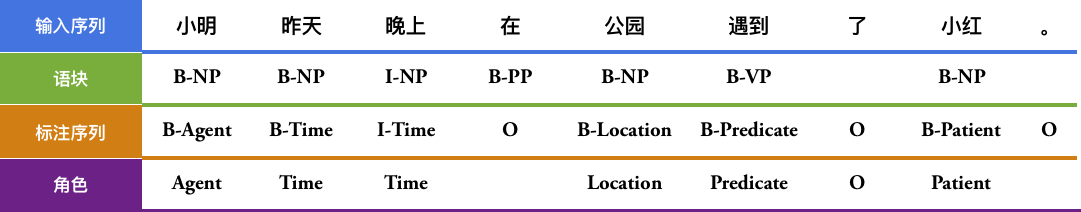Figure 2. Example of BIO labeling method

As can be seen from the above example, it is a relatively simple process to directly get the semantic roles labeling result of the argument according to the sequence labeling result. This simplicity is reflected in: (1) relying on shallow syntactic analysis, reducing the requirements and difficulty of syntactic analysis; (2) there is no candidate argument to pruning in this step; (3) the identification and labeling of arguments are realized at the same time. This integrated approach to arguments identification and labeling simplifies the process, reduces the risk of error accumulation, and often achieves better results.

Similar to the block-based SRL method, in this tutorial we also regard the SRL as a sequence labeling problem. The difference is that we only rely on input text sequences, without relying on any additional syntax analysis results or complex artificial features. And constructing an end-to-end learning SRL system by using deep neural networks. Let’s take the public data set of the SRL task in the CoNLL-2004 and CoNLL-2005 Shared Tasks task as an example to practice the following tasks. Giving a sentence and a predicate in this sentence, through the way of sequence labeling, find the arguments corresponding to the predicate from the sentence, and mark their semantic roles.

## Model Overview¶

Recurrent Neural Network is an important model for modeling sequences. It is widely used in natural language processing tasks. Unlike the feed-forward neural network, the RNN is able to handle the contextual correlation between inputs. LSTM is an important variant of RNN that is commonly used to learn the long-range dependencies contained in long sequences. We have already introduced in Sentiment Analysis, in this article we still use LSTM to solve the SRL problem.

### Stacked Recurrent Neural Network¶

The deep network helps to form hierarchical features, and the upper layers of the network form more complex advanced features based on the primary features that have been learned in the lower layers. Although the LSTM is expanded along the time axis and is equivalent to a very “deep” feedforward network. However, since the LSTM time step parameters are shared, the mapping of the $t-1$ time state to the time of $t$ always passes only one non-linear mapping. It means that the modeling of state transitions by single-layer LSTM is “shallow”. Stacking multiple LSTM units, making the output of the previous LSTM$t$ time as the input of the next LSTM unit $t$ time, helps us build a deep network. We call it the first version of the stack ecurrent neural networks. Deep networks improve the ability of models to fit complex patterns and better model patterns across different time steps.

However, training a deep LSTM network is not an easy task. Stacking multiple LSTM cells in portrait orientation may encounter problems with the propagation of gradients in the longitudinal depth. Generally, stacking 4 layers of LSTM units can be trained normally. When the number of layers reaches 4~8 layers, performance degradation will occur. At this time, some new structures must be considered to ensure the gradient is transmitted vertically and smoothly. This is a problem that must be solved in training a deep LSTM networks. We can learn from LSTM to solve one of the tips of the “gradient disappearance and gradient explosion” problem: there is no nonlinear mapping on the information propagation route of Memory Cell, and neither gradient decay nor explosion when the gradient propagates back. Therefore, the deep LSTM model can also add a path that ensures smooth gradient propagation in the vertical direction.

The operation performed by an LSTM unit can be divided into three parts: (1) Input-to-hidden: Each time step input information $x$ will first pass through a matrix map and then as a forgetting gate, input gate, memory unit, output gate’s input. Note that this mapping does not introduce nonlinear activation; (2) Hidden-to-hidden: this step is the main body of LSTM calculation, including forgotten gate, input gate, memory unit update, output gate calculation; (3) hidden-to-output: usually simple to activate the hidden layer vector. On the basis of the first version of the stack network, we add a new path: in addition to the previous LSTM output, the mapping of the input of the previous LSTM to the hidden layer is used as a new input. and a new input is added. At the same time, add a linear map to learn a new transform.

Figure 3 is a schematic structural diagram of a finally obtained stack recurrent neural network.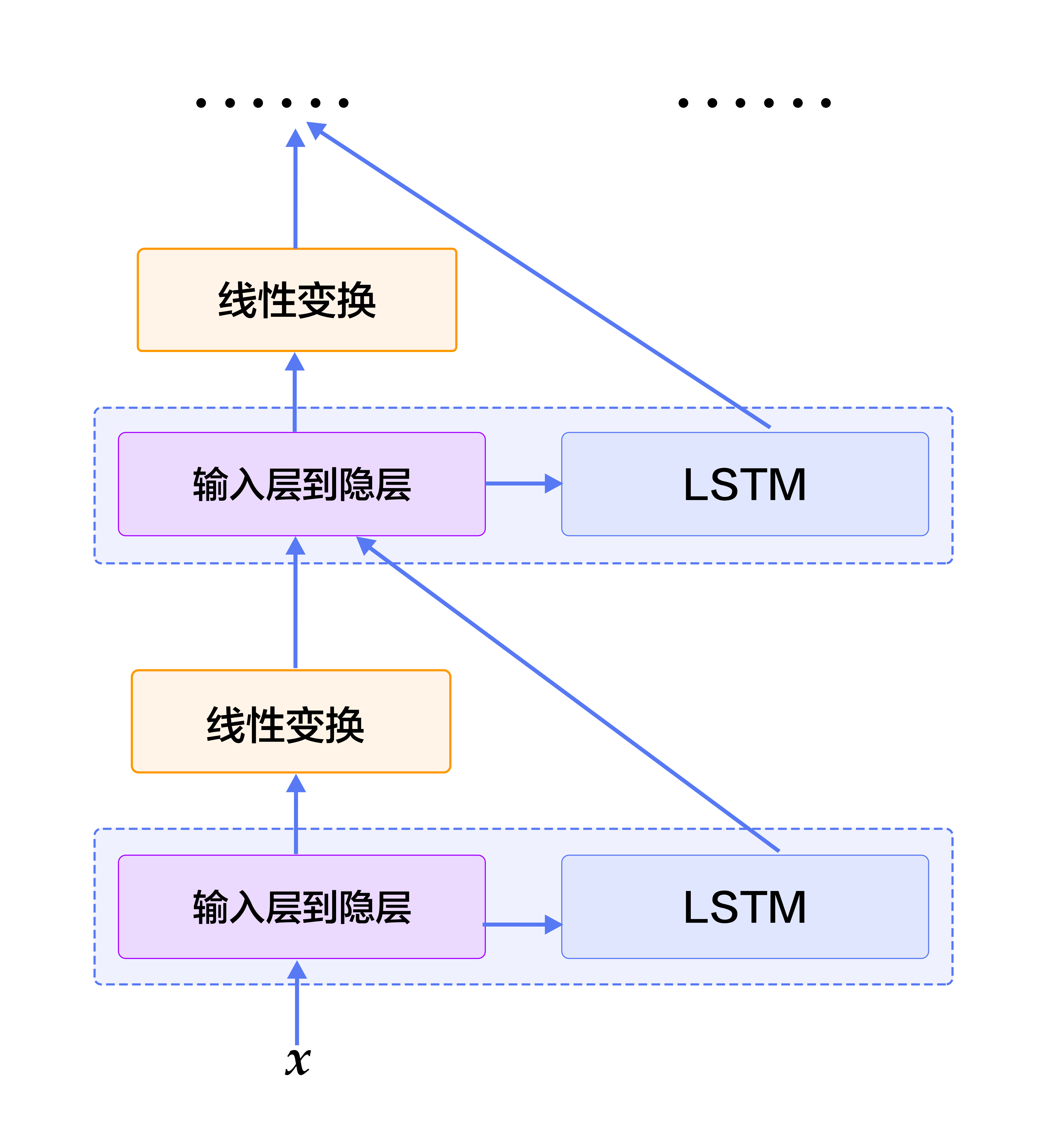Figure 3. Schematic diagram of stack-based recurrent neural network based on LSTM

### Bidirectional Recurrent Neural Network¶

In LSTM, the hidden layer vector at the time of $t$ encodes all input information until the time of $t$. The LSTM at $t$ can see the history, but cannot see the future. In most natural language processing tasks, we almost always get the whole sentence. In this case, if you can get future information like the historical information, it will be of great help to the sequence learning task.

In order to overcome this shortcoming, we can design a bidirectional recurrent network unit, which is simple and straightforward: make a small modification to the stack recurrent neural network of the previous section, stack multiple LSTM units, and let each layer of LSTM units learn the output sequence of the previous layer in the order of forward, reverse, forward …… So, starting from layer 2, our LSTM unit will always see historical and future information at $t$. Figure 4 is a schematic diagram showing the structure of a bidirectional recurrent neural network based on LSTM.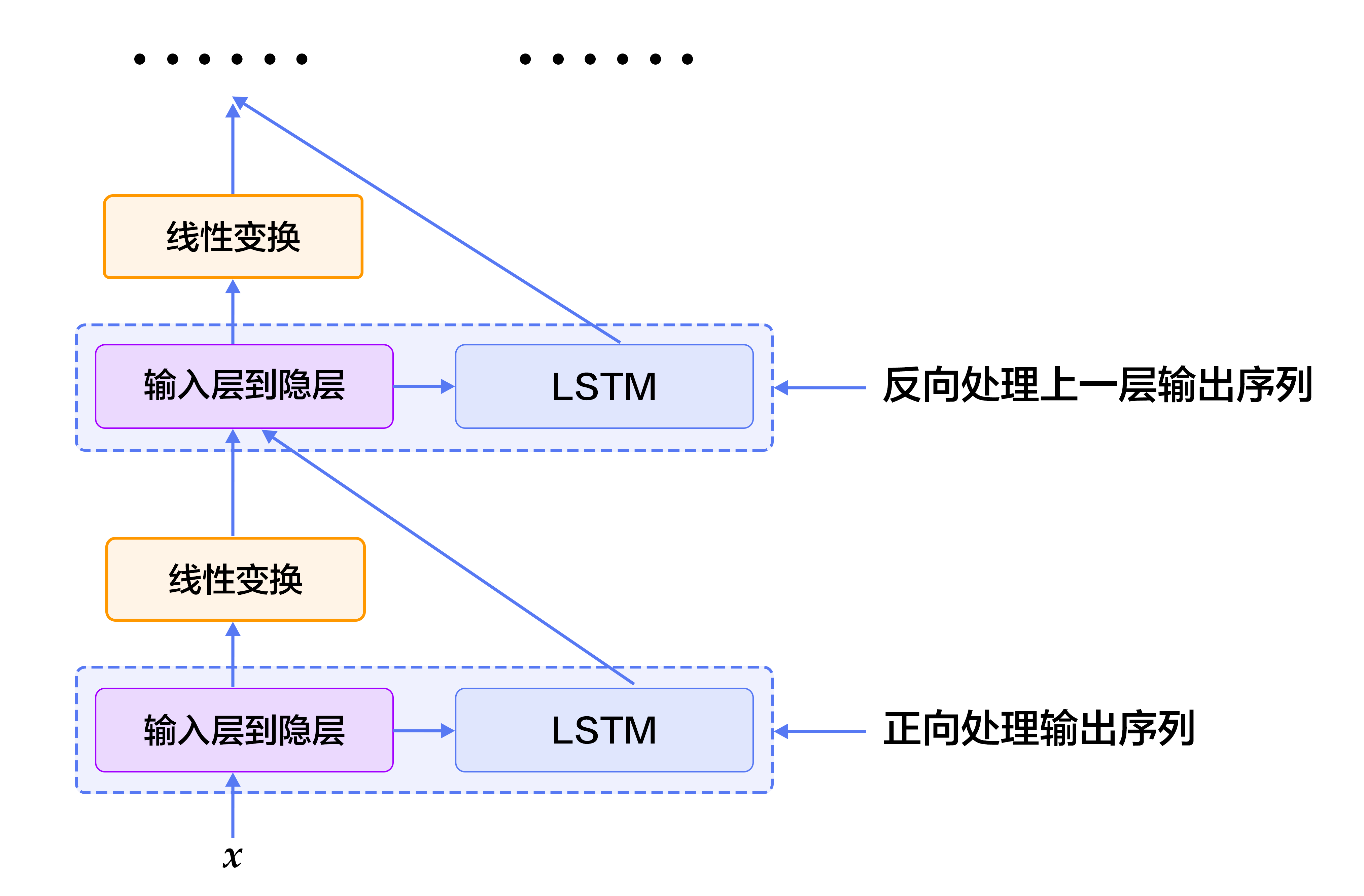Figure 4. Schematic diagram of a bidirectional recurrent neural network based on LSTM

It should be noted that this bidirectional RNN structure is not the same as the bidirectional RNN structure used by Bengio etc in machine translation tasks[3, 4] Another bidirectional recurrent neural network will be introduced in the following Machine Translation task.

### Conditional Random Field¶

The idea of ​​using a neural network model to solve a problem usually is: the front-layer network learns the feature representation of the input, and the last layer of the network completes the final task based on the feature. In the SRL task, the feature representation of the deep LSTM network learns input. Conditional Random Filed (CRF) completes the sequence labeling on th basis of features at the end of the entire network.

CRF is a probabilistic structural model, which can be regarded as a probabilistic undirected graph model. Nodes represent random variables and edges represent probability dependencies between random variables. In simple terms, CRF learns the conditional probability $P(X|Y)$, where $X = (x_1, x_2, … , x_n)$ is the input sequence, $Y = (y_1, y_2, …, y_n$ is a sequence of tokens; the decoding process is given the $X$ sequence to solve the $Y$ sequence with the largest $P(Y|X)$, that is $Y^* = \mbox{arg max}_{Y} P( Y | X)$.

The sequence labeling task only needs to consider that both the input and the output are a linear sequence. And since we only use the input sequence as a condition and do not make any conditional independent assumptions, there is no graph structure between the elements of the input sequence. In summary, the CRF defined on the chain diagram shown in Figure 5 is used in the sequence labeling task, which is called Linear Chain Conditional Random Field.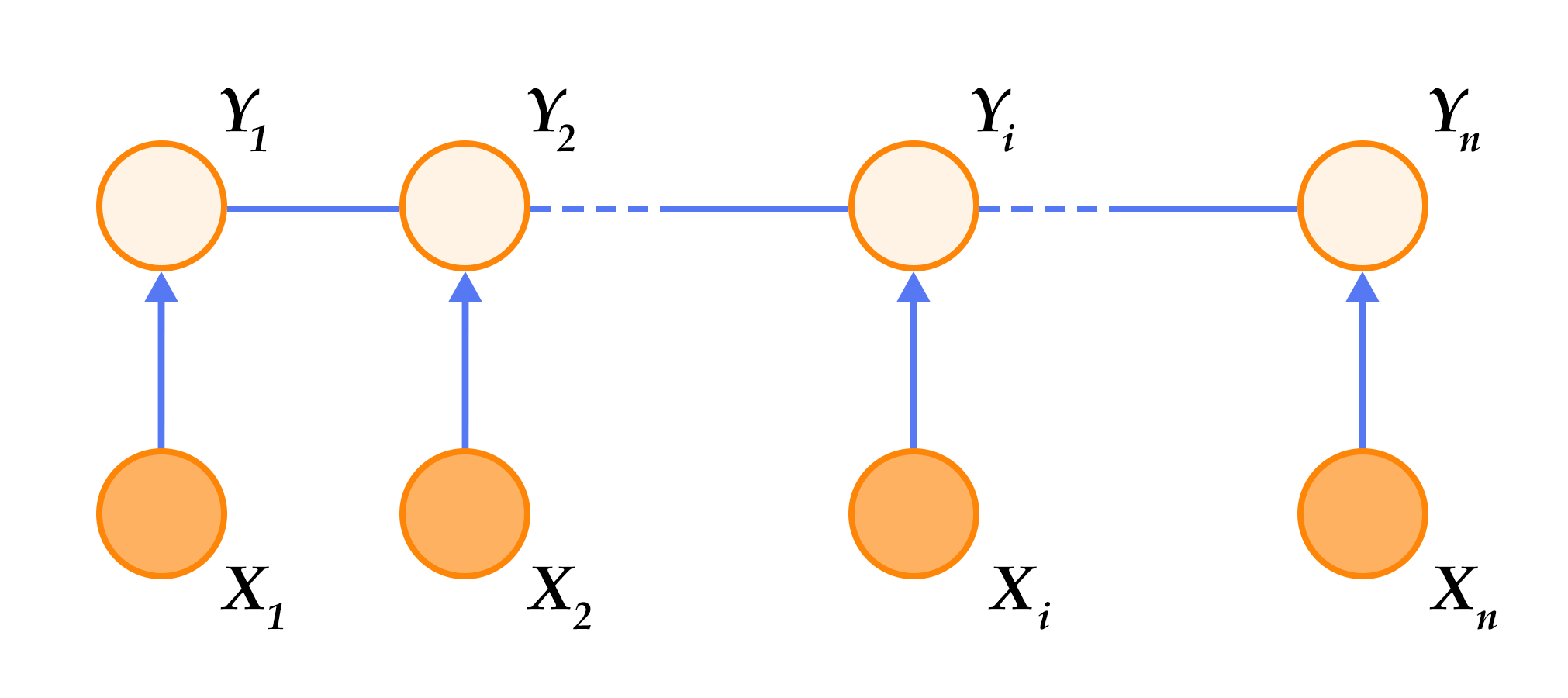Figure 5. Linear chain conditional random field used in sequence labeling tasks

According to the factorization theorem on the linear chain condition random field , the probability of a particular tag sequence $Y$ can be defined as given in the observation sequence $X$:

$$p(Y | X) = \frac{1}{Z(X)} \text{exp}\left(\sum_{i=1}^{n}\left(\sum_{j}\lambda_{ j}t_{j} (y_{i - 1}, y_{i}, X, i) + \sum_{k} \mu_k s_k (y_i, X, i)\right)\right)$$

Where $Z(X)$ is the normalization factor, and $t_j$ is the feature function defined on the edge, depending on the current and previous position, which called the transition feature. It represents the transition probability of the input sequence $X$ and its labeling sequence marked at the $i$ and $i - 1$ positions. $s_k$ is a feature function defined on the node, called a state feature, which depends on the current position. It represents the probability of marking for the observation sequence $X$ and its $i$ position. $\lambda_j$ and $\mu_k$ are the weights corresponding to the transfer feature function and the state feature function respectively. In fact, $t$ and $s$ can be represented in the same mathematical form, and the transfer feature and state are summed at each position $i$: $f_{k}(Y, X) = \sum_{i =1}^{n}f_k({y_{i - 1}, y_i, X, i})$. Calling $f$ collectively as a feature function, so $P(Y|X)$ can be expressed as:

$$p(Y|X, W) = \frac{1}{Z(X)}\text{exp}\sum_{k}\omega_{k}f_{k}(Y, X)$$

$\omega$ is the weight corresponding to the feature function and is the parameter to be learned by the CRF model. During training, for a given input sequence and the corresponding set of markup sequences $D = \left[(X_1, Y_1), (X_2 , Y_2) , … , (X_N, Y_N)\right]$ , by regularizing the maximum likelihood estimation to solve the following optimization objectives:

$$\DeclareMathOperator*{\argmax}{arg,max} L(\lambda, D) = - \text{log}\left(\prod_{m=1}^{N}p(Y_m|X_m, W )\right) + C \frac{1}{2}\lVert W\rVert^{2}$$

This optimization objectives can be solved by the back propagation algorithm together with the entire neural network. When decoding, for a given input sequence $X$, the output sequence $\bar{Y}$ of maximizing the conditional probability $\bar{P}(Y|X)$ by the decoding algorithm (such as: Viterbi algorithm, Beam Search).

### Deep bidirectional LSTM (DB-LSTM) SRL model¶

In the SRL task, the input is “predicate” and “a sentence”. The goal is to find the argument of the predicate from this sentence and mark the semantic role of the argument. If a sentence contains $n$ predicates, the sentence will be processed for $n$ times. One of the most straightforward models is the following:

1. Construct the input;

• Input 1 is the predicate and 2 is the sentence

• Extend input 1 to a sequence as long as input 2, expressed by one-hot mode;

1. The predicate sequence and sentence sequence of the one-hot format are converted into a sequence of word vectors represented by real vectors through a vocabulary;

2. The two word vector sequences in step 2 are used as input of the bidirectional LSTM to learn the feature representation of the input sequence;

3. The CRF takes the features learned in the model in step 3 as input, and uses the tag sequence as the supervised signal to implement sequence labeling;

You can try this method. Here, we propose some improvements that introduce two simple features that are very effective in improving system performance:

• Predicate’s context: In the above method, only the word vector of the predicate is used to express all the information related to the predicate. This method is always very weak, especially if the predicate appears multiple times in the sentence, it may cause certain ambiguity. From experience, a small segment of several words before and after the predicate can provide more information to help resolve ambiguity. So, we add this kind of experience to the model, and extract a “predicate context” fragment for each predicate, that is, a window fragment composed of $n$ words before and after the predicate;

• Predicate context area’s tag: Introduces a 0-1 binary variable for each word in the sentence, which indicats whether they are in the “predicate context” fragment;

The modified model is as follows (Figure 6 is a schematic diagram of the model structure with a depth of 4):

1. Construct input

• Input 1 is a sentence sequence, input 2 is a predicate sequence, input 3 is a predicate context, and $n$ words before and after the predicate are extracted from the sentence to form a predicate context, which represented by one-hot. Input 4 is a predicate context area which marks whether each word in the sentence is in the context of the predicate;

• Extend the input 2~3 to a sequence as long as the input 1;

1. Input 1~4 are converted into a sequence of word vectors represented by real vectors in vocabulary; where inputs 1 and 3 share the same vocabulary, and inputs 2 and 4 each have their own vocabulary;

2. The four word vector sequences in step 2 are used as input to the bidirectional LSTM model; the LSTM model learns the feature representation of the input sequence to obtain a new feature representation sequence;

3. The CRF takes the features learned in step 3 of the LSTM as input, and uses the marked sequence as the supervised signal to complete the sequence labeling;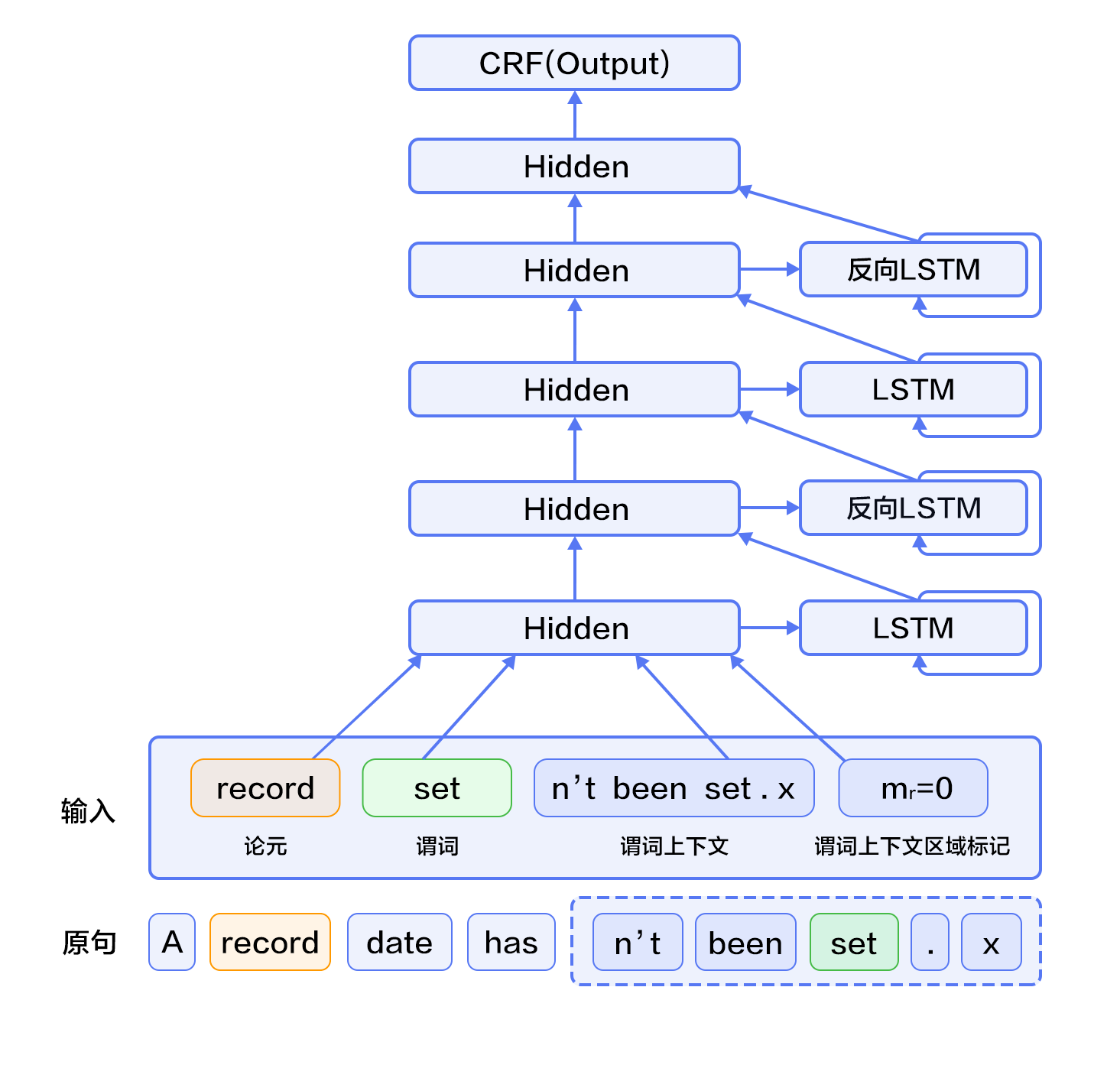Figure 6. Deep bidirectional LSTM model on the SRL task

## Data Introduction¶

In this tutorial, We use the data set opened by the CoNLL 2005 SRL task as an example. It is important to note that the training set and development set of the CoNLL 2005 SRL task are not free for public after the competition. Currently, only the test set is available, including 23 in the Wall Street Journal and 3 in the Brown corpus. In this tutorial, we use the WSJ data in the test set to solve the model for the training set. However, since the number of samples in the test set is far from enough, if you want to train an available neural network SRL system, consider paying for the full amount of data.

The original data also includes a variety of information such as part-of-speech tagging, named entity recognition, and syntax parse tree. In this tutorial, we use the data in the test.wsj folder for training and testing, and only use the data under the words folder (text sequence) and the props folder (labeled results). The data directories used in this tutorial are as follows:

conll05st-release/
└── test.wsj
├── props # Label result
└── words # Input text sequence


The labeling information is derived from the labeling results of Penn TreeBank and PropBank. The label of the PropBank labeling result is different from the labeling result label we used in the first example of the article, but the principle is the same. For the description of the meaning of the labeling result label, please refer to the paper .

The raw data needs to be preprocessed in order to be processed by PaddlePaddle. The preprocessing includes the following steps:

1. Combine text sequences and tag sequences into one record;

2. If a sentence contains $n$ predicates, the sentence will be processed for $n$ times, becoming a $n$ independent training sample, each sample with a different predicate;

3. Extract the predicate context and construct the predicate context area tag;

4. Construct a tag represented by the BIO method;

5. Get the integer index corresponding to the word according to the dictionary.

After the pre-processing is completed, a training sample data contains 9 fields, namely: sentence sequence, predicate, predicate context (accounting for 5 columns), predicate context area tag, and labeling sequence. The following table is an example of a training sample.

Sentence Sequence Predicate Predicate Context (Window = 5) Predicate Context Area Tag Label Sequence
A set n't been set . × 0 B-A1
record set n't been set . × 0 I-A1
date set n't been set . × 0 I-A1
has set n't been set . × 0 O
n't set n't been set . × 1 B-AM-NEG
been set n't been set . × 1 O
set set n't been set . × 1 B-V
. set n't been set . × 1 O

In addition to the data, we also provide the following resources:

File Name Description
word_dict Input a dictionary of sentences for a total of 44068 words
label_dict Tag dictionary, total 106 tags
predicate_dict Dictionary of predicates, totaling 3162 words
emb A trained vocabulary, 32-dimensional

We trained a language model on English Wikipedia to get a word vector to initialize the SRL model. During the training of the SRL model, the word vector is no longer updated. For the language model and word vector, refer to Word Vector for this tutorial. The corpus of our training language model has a total of 995,000,000 tokens, and the dictionary size is controlled to 4,900,000 words. CoNLL 2005 training corpus 5% of this word is not in 4900,000 words, we have seen them all unknown words, with <unk> representation.

Get the dictionary and print the dictionary size:

from __future__ import print_function

import math, os
import numpy as np
import paddle
import paddle.dataset.conll05 as conll05
import paddle.fluid as fluid
import six
import time

with_gpu = os.getenv('WITH_GPU', '0') != '0'

word_dict, verb_dict, label_dict = conll05.get_dict()
word_dict_len = len(word_dict)
label_dict_len = len(label_dict)
pred_dict_len = len(verb_dict)

print('word_dict_len: ', word_dict_len)
print('label_dict_len: ', label_dict_len)
print('pred_dict_len: ', pred_dict_len)


## Model Configuration¶

• Define input data dimensions and model hyperparameters.

mark_dict_len = 2    # The dimension of the context area flag, which is a 0-1 2 value feature, so the dimension is 2
word_dim = 32        # Word vector dimension
mark_dim = 5         # The predicate context area is mapped to a real vector by the vocabulary, which is the adjacent dimension
hidden_dim = 512     # LSTM Hidden Layer Vector Dimensions : 512 / 4
depth = 8            # depth of stack LSTM
mix_hidden_lr = 1e-3 # Basic learning rate of fundamental_chain_crf layer

IS_SPARSE = True     # Whether to update embedding in sparse way
PASS_NUM = 10        # Training epoches
BATCH_SIZE = 10      # Batch size

embedding_name = 'emb'


It should be specially noted that the parameter hidden_dim = 512 actually specifies the dimension of the LSTM hidden layer’s vector is 128. For this, please refer to the description of dynamic_lstm in the official PaddlePaddle API documentation.

• As is mentioned above, we use the trained word vector based on English Wikipedia to initialize the embedding layer parameters of the total six features of the sequence input and predicate context, which are not updated during training.

#Here load the binary parameters saved by PaddlePaddle
def load_parameter(file_name, h, w):
with open(file_name, 'rb') as f:
f.read(16)  # skip header.
return np.fromfile(f, dtype=np.float32).reshape(h, w)


## Training Model¶

• We train according to the network topology and model parameters. We also need to specify the optimization method when constructing. Here we use the most basic SGD method (momentum is set to 0), and set the learning rate, regularition, and so on.

Define hyperparameters for the training process

use_cuda = False #Execute training on cpu
save_dirname = "label_semantic_roles.inference.model" #The model parameters obtained by training are saved in the file.
is_local = True


### Data input layer definition¶

Defines the format of the model input features, including the sentence sequence, the predicate, the five features of the predicate context, and the predicate context area flags.

# Sentence sequences
word = fluid.data(
name='word_data', shape=[None, 1], dtype='int64', lod_level=1)

# predicate
predicate = fluid.data(
name='verb_data', shape=[None, 1], dtype='int64', lod_level=1)

# predicate context's 5 features
ctx_n2 = fluid.data(
name='ctx_n2_data', shape=[None, 1], dtype='int64', lod_level=1)
ctx_n1 = fluid.data(
name='ctx_n1_data', shape=[None, 1], dtype='int64', lod_level=1)
ctx_0 = fluid.data(
name='ctx_0_data', shape=[None, 1], dtype='int64', lod_level=1)
ctx_p1 = fluid.data(
name='ctx_p1_data', shape=[None, 1], dtype='int64', lod_level=1)
ctx_p2 = fluid.data(
name='ctx_p2_data', shape=[None, 1], dtype='int64', lod_level=1)

# Predicate conotext area flag
mark = fluid.data(
name='mark_data', shape=[None, 1], dtype='int64', lod_level=1)


### Defining the network structure¶

First pre-train and define the model input layer

#pre-training predicate and predicate context area flags
predicate_embedding = fluid.embedding(
input=predicate,
size=[pred_dict_len, word_dim],
dtype='float32',
is_sparse=IS_SPARSE,
param_attr='vemb')

mark_embedding = fluid.embedding(
input=mark,
size=[mark_dict_len, mark_dim],
dtype='float32',
is_sparse=IS_SPARSE)

#Sentence sequences and predicate context 5 features then pre-trained
word_input = [word, ctx_n2, ctx_n1, ctx_0, ctx_p1, ctx_p2]
#Because word vector is pre-trained, no longer training embedding table,
# The trainable's parameter attribute set to False prevents the embedding table from being updated during training
emb_layers = [
fluid.embedding(
size=[word_dict_len, word_dim],
input=x,
param_attr=fluid.ParamAttr(
name=embedding_name, trainable=False)) for x in word_input
]
# Pre-training results for adding predicate and predicate context area tags
emb_layers.append(predicate_embedding)
emb_layers.append(mark_embedding)


Define eight LSTM units to learn all input sequences in “forward/reverse” order.

# A total of 8 LSTM units are trained, each unit is oriented from left to right or right to left.
# Determined by the parameter is_reverse
# First stack structure
hidden_0_layers = [
fluid.layers.fc(input=emb, size=hidden_dim, act='tanh')
for emb in emb_layers
]

hidden_0 = fluid.layers.sums(input=hidden_0_layers)

lstm_0 = fluid.layers.dynamic_lstm(
input=hidden_0,
size=hidden_dim,
candidate_activation='relu',
gate_activation='sigmoid',
cell_activation='sigmoid')

# Stack L-LSTM and R-LSTM with directly connected sides
input_tmp = [hidden_0, lstm_0]

# remaining stack structure
for i in range(1, depth):
mix_hidden = fluid.layers.sums(input=[
fluid.layers.fc(input=input_tmp, size=hidden_dim, act='tanh'),
fluid.layers.fc(input=input_tmp, size=hidden_dim, act='tanh')
])

lstm = fluid.layers.dynamic_lstm(
input=mix_hidden,
size=hidden_dim,
candidate_activation='relu',
gate_activation='sigmoid',
cell_activation='sigmoid',
is_reverse=((i % 2) == 1))

input_tmp = [mix_hidden, lstm]

# Fetch the output of the last stack LSTM and the input of this LSTM unit to the hidden layer mapping,
# Learn the state feature of CRF after a fully connected layer maps to the dimensions of the tags dictionary
feature_out = fluid.layers.sums(input=[
fluid.layers.fc(input=input_tmp, size=label_dict_len, act='tanh'),
fluid.layers.fc(input=input_tmp, size=label_dict_len, act='tanh')
])

# tag/label sequence
target = fluid.data(
name='target', shape=, dtype='int64', lod_level=1)

# Learning CRF transfer features
crf_cost = fluid.layers.linear_chain_crf(
input=feature_out,
label=target,
param_attr=fluid.ParamAttr(
name='crfw', learning_rate=mix_hidden_lr))

avg_cost = fluid.layers.mean(crf_cost)

# Use the most basic SGD optimization method (momentum is set to 0)
sgd_optimizer = fluid.optimizer.SGD(
learning_rate=fluid.layers.exponential_decay(
learning_rate=0.01,
decay_steps=100000,
decay_rate=0.5,
staircase=True))

sgd_optimizer.minimize(avg_cost)



The data introduction section mentions the payment of the CoNLL 2005 training set. Here we use the test set training for everyone to learn. Conll05.test() produces one sample every time, containing 9 features, then shuffle and after batching as the input for training.

crf_decode = fluid.layers.crf_decoding(
input=feature_out, param_attr=fluid.ParamAttr(name='crfw'))

train_data = fluid.io.batch(
fluid.io.shuffle(
paddle.dataset.conll05.test(), buf_size=8192),
batch_size=BATCH_SIZE)

place = fluid.CUDAPlace(0) if use_cuda else fluid.CPUPlace()


The corresponding relationship between each data and data_layer is specified by the feeder. The following feeder indicates that the data_layer corresponding to the 0th column of the data generated by conll05.test() is word.

feeder = fluid.DataFeeder(
feed_list=[
word, ctx_n2, ctx_n1, ctx_0, ctx_p1, ctx_p2, predicate, mark, target
],
place=place)
exe = fluid.Executor(place)


Start training

main_program = fluid.default_main_program()

exe.run(fluid.default_startup_program())
embedding_param = fluid.global_scope().find_var(
embedding_name).get_tensor()
embedding_param.set(
load_parameter(conll05.get_embedding(), word_dict_len, word_dim),
place)

start_time = time.time()
batch_id = 0
for pass_id in six.moves.xrange(PASS_NUM):
for data in train_data():
cost = exe.run(main_program,
feed=feeder.feed(data),
fetch_list=[avg_cost])
cost = cost

if batch_id % 10 == 0:
print("avg_cost: " + str(cost))
if batch_id != 0:
print("second per batch: " + str((time.time(
) - start_time) / batch_id))
# Set the threshold low to speed up the CI test
if float(cost) < 60.0:
if save_dirname is not None:
fluid.io.save_inference_model(save_dirname, [
'word_data', 'verb_data', 'ctx_n2_data',
'ctx_n1_data', 'ctx_0_data', 'ctx_p1_data',
'ctx_p2_data', 'mark_data'
], [feature_out], exe)
break

batch_id = batch_id + 1


## Model Application¶

After completing the training, the optimal model needs to be selected according to a performance indicator we care about. You can simply select the model with the least markup error on the test set. We give an example of using a trained model for prediction as follows.

First set the parameters of the prediction process

use_cuda = False #predict on cpu
save_dirname = "label_semantic_roles.inference.model" #call trained model for prediction

place = fluid.CUDAPlace(0) if use_cuda else fluid.CPUPlace()
exe = fluid.Executor(place)


Set the input, use LoDTensor to represent the input word sequence, where the shape of each word’s base_shape is , because each word is represented by an id. If the length-based LoD is [[3, 4, 2]], which is a single-layer LoD, then the constructed LoDTensor contains three sequences which their length are 3, 4, and 2.

Note that LoD is a list of lists.

lod = [[3, 4, 2]]
base_shape = 

# Construct fake data as input, the range of random integer numbers is [low, high]
word = fluid.create_random_int_lodtensor(
lod, base_shape, place, low=0, high=word_dict_len - 1)
pred = fluid.create_random_int_lodtensor(
lod, base_shape, place, low=0, high=pred_dict_len - 1)
ctx_n2 = fluid.create_random_int_lodtensor(
lod, base_shape, place, low=0, high=word_dict_len - 1)
ctx_n1 = fluid.create_random_int_lodtensor(
lod, base_shape, place, low=0, high=word_dict_len - 1)
ctx_0 = fluid.create_random_int_lodtensor(
lod, base_shape, place, low=0, high=word_dict_len - 1)
ctx_p1 = fluid.create_random_int_lodtensor(
lod, base_shape, place, low=0, high=word_dict_len - 1)
ctx_p2 = fluid.create_random_int_lodtensor(
lod, base_shape, place, low=0, high=word_dict_len - 1)
mark = fluid.create_random_int_lodtensor(
lod, base_shape, place, low=0, high=mark_dict_len - 1)


Using fluid.io.load_inference_model to load inference_program, feed_target_names is the name of the model’s input variable, and fetch_targets is the predicted object.

[inference_program, feed_target_names,
fetch_targets] = fluid.io.load_inference_model(save_dirname, exe)


Construct the feed dictionary {feed_target_name: feed_target_data}, where the results are a list of predicted targets

assert feed_target_names == 'word_data'
assert feed_target_names == 'verb_data'
assert feed_target_names == 'ctx_n2_data'
assert feed_target_names == 'ctx_n1_data'
assert feed_target_names == 'ctx_0_data'
assert feed_target_names == 'ctx_p1_data'
assert feed_target_names == 'ctx_p2_data'
assert feed_target_names == 'mark_data'


Execute prediction

results = exe.run(inference_program,
feed={
feed_target_names: word,
feed_target_names: pred,
feed_target_names: ctx_n2,
feed_target_names: ctx_n1,
feed_target_names: ctx_0,
feed_target_names: ctx_p1,
feed_target_names: ctx_p2,
feed_target_names: mark
},
fetch_list=fetch_targets,
return_numpy=False)


Output result

print(results.lod())
np_data = np.array(results)
print("Inference Shape: ", np_data.shape)


## Conclusion¶

Labeling semantic roles is an important intermediate step in many natural language understanding tasks. In this tutorial, we take the label semantic roles task as an example to introduce how to use PaddlePaddle for sequence labeling tasks. The model presented in the tutorial comes from our published paper . Since the training data for the CoNLL 2005 SRL task is not currently fully open, only the test data is used as an example in the tutorial. In this process, we hope to reduce our reliance on other natural language processing tools. We can use neural network data-driven, end-to-end learning capabilities to get a model that is comparable or even better than traditional methods. In the paper, we confirmed this possibility. More information and discussion about the model can be found in the paper.